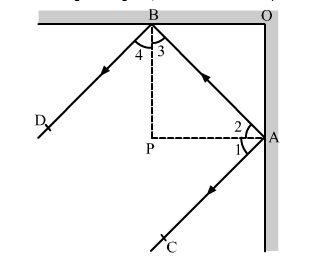# In the given figure, m and n are two plane mirrors perpendicular to each other.

Question:

In the given figure, m and n are two plane mirrors perpendicular to each other. Show that the incident ray CA is parallel to the reflected ray BD.Solution:

AP is normal to the plane mirror OA and BP is normal to the plane mirror OB.

It is given that the two plane mirrors are perpendicular to each other.

Therefore, BP || OA and AP || OB.

So, BP ⊥ AP       (OA ⊥ OB)

⇒ ∠APB = 90°    .....(1)

In ∆APB,

​∠2 + ∠3 + ∠APB = 180°      (Angle sum property)

∴ ∠2 + ∠3 + 90° = 180°       [Using (1)]

⇒ ∠2 + ∠3 = 180° − 90° = 90°

⇒ 2∠2 + 2∠3 = 2 × 90° = 180°        .....(2)

By law of reflection, we have

∠1 = ∠2  and ∠3 = ∠4                     .....(3)       (Angle of incidence = Angle of reflection)

From (2) and (3), we have

∠1 + ∠2 + ∠3 + ∠4 = 180°

⇒ ∠BAC + ∠ABD = 180°            (∠1 + ∠2 = ∠BAC and ∠3 + ∠4 = ∠ABD)

Thus, the lines CA and BD are intersected by a transversal AB such that the interior angles on the same side of the transversal are supplementary.

∴ CA || BD